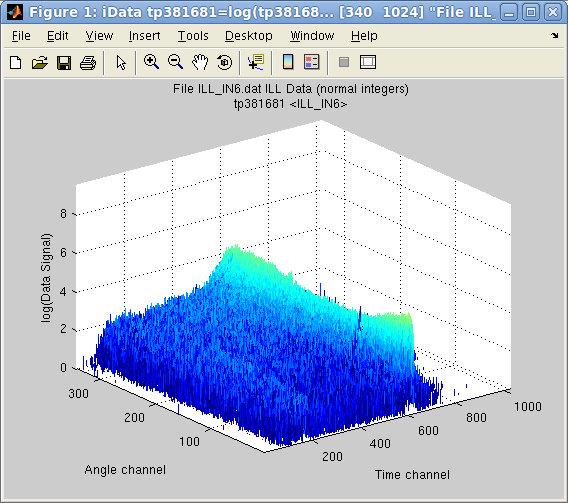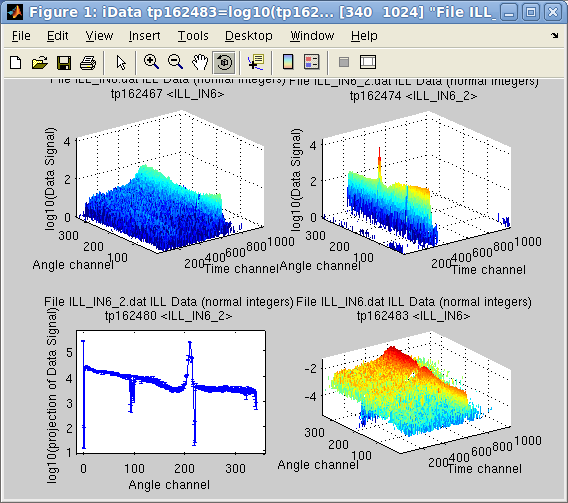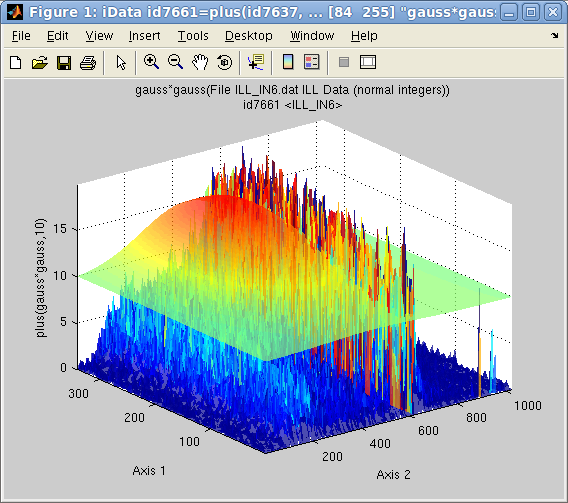# iFit: a quick start/tutorial

Commands we use in this page: iData, doc, plot, subplot, fits, saveas

In this document, we demonstrate shortly how to use the iFit library, which mainly manipulates iData objects. We focus on commands.

The object iFit is to:
• import transparently data files, whatever be their format [Load]
• organize the data files contents, and in particular define their axes if needed [iData axes and aliases]
• display/plot the data [Plot]
• apply mathematical operations [Math]
• fit multi parameter models onto data sets [Fit]
• save results transparently into some common scientific formats [Save]
Each of these tasks has dedicated, detailed, documentation, as shown in links above.

### Starting

Currently, iFit depends on a Matlab installation, and we assume that iFit has been installed properly before. If not so, please refer to the Install page. Then we start Matlab, and declare the iFit library location.
`\$ matlab                              % start Matlab (or click its icon)>> addpath(genpath('/path/to/iFit'))  % declare the iFit library location so that we can use it>> doc(iData)                         % open the iFit/iData documentation (if you feel like reading it)`
We are ready to work. For the purpose of this tutorial, we shall handle some 1D and 2D example data sets.

Let's import a file from the iFit example data files. This is done by converting a character string, assumed to be a file name, as an iData object:
`>> a = iData([ ifitpath 'Data/ILL_IN6.dat' ]);`
which is a neutron scattering signal by a liquid, collected by the instrument IN6@ILL. Importation also works across the Internet with http:// and ftp:// file specifications, as well as with compressed contents. A file selector pops-up when the file name is given as '' (empty string). The result of the importation is an iData object:
`>> aa =  iData object:    [Tag]     [Dimension]                           [Title] [Last command]          [Label] tp574029     [340  1024] 'File ILL_IN6.dat ILL Data (n...' tp574029=load(iData,...  `

### Axes and Aliases

We may inquire the contents of that object with the get method
`>> get(a)>> disp(a)`
which interestingly indicates that a Signal has been defined (by default the largest numerical block in the object). This Signal is an Alias which points to the Data area of the object, and more specifically in this case to the Data.IIIIIIIIIIIIIIIIIIIIIIIIIIIII_13 section. The naming of the section has been determined automatically from the file comments. The Signal may be re-assigned any time, as well as possible axes attached. This common mechanism uses the Alias feature of the iData object.

The axes of the IN6 signal are related to a neutron detection time, and an angle w.r.t the direct beam. We thus define the labels for these two axes:
`>> xlabel(a, 'Time channel'); % 2nd axis (columns)>> ylabel(a, 'Angle channel');% 1st axis (rows)`
which depends on the convention used to represent matrices. In Matlab, matrices are stored in column-major order, that is first axis is a row, within which elements are stored along columns: Signal(row, column). As the 'X' axis usually refers to the horizontal one for a 2D signal (matrix), it thus relates to columns, that is the second dimension. The 'Y' axis is vertical, and relates to rows, that is first dimension.

Some of the importable file formats make it easy to find the Signal, and its corresponding axes. But other formats may contain many numerical blocks within which none appears de facto as The Signal. This is the same for axes. Then, the Signal and axes may be redefined in order to match the user requirements.

The concepts of Alias, Signal and Axes are of major importance, especially as they impact the way mathematical operators apply onto objects, and their rendering in plots.

More about iData object, alias, axes, Signal

### Display objectsIn order to display the object contents, that is its Signal as a function of axes, possibly weighted by a Monitor, a single command can be used independently of the data set dimensionality.
`>> plot(a)`
but as, in this case, the Signal contains a single large value well above the interesting stuff, we may simply ask to display the object in log10 scale.
`>> plot(log10(a))`
It is also possible to display more than one object overlayed onto a single plot, or side by side in the same window.

### Mathematical operators

A large set of mathematical operators can be applied on iData objects, and may be used to correct/analyze the Signal. Whenever the Signal or the axes are changed (by value or definition), the operator result will change accordingly. This way it is possible to define a series of operations for data processing, independently of the data files representation.

Let's import a second IN6 data file, and set its axes labels as for the first object.
`>> b = iData([ ifitpath 'Data/ILL_IN6_2.dat' ]);>> xlabel(b, 'Time channel'); % 2nd axis (columns)>> ylabel(b, 'Angle channel');% 1st axis (rows)`Then we may obtain the projection of this object onto the first dimension 'Angle', as a new object. Then we normalize the first object 'a' by this projection, and plot the two initial data file contents, the projection of the second onto the Angle axis, and the normalization of the first object by this projection.
`>> c = camproj(b)>> subplot(log10([a b c a./c ]))`
In the latter command, the log10 applies onto the vector of the 4 objects. Also, the division is made element by element with the ./ operator and the projection c (1D signal) is extended to a 2D object in order to perform the division.

All binary operators can cope with objects that may have partly overlapping axes, possibly with different binning, and of different dimensionality. This is done transparently.

Also, the Error estimates  associated to the Signal are propagated, assuming distributions are Gaussians.

### Advanced Math operators: interpolation, concatenating, fitting

The interpolation of an object onto a new set of axes is done transparently, whatever be the object dimensionality. An object rebinning is obtained by interpolating the object on its own axes, or a sub-set of it:
`>> d = interp(a, 1/4);    % rebin by dividing the length of axes by 4 `Adjusting a function-model onto an object is easy, in order to determine the best parameter set that describes the Signal/Monitor. The fitting procedure makes sure that the model matches the object dimensionality, and extends it if needed.
`>> d(find(d>200))=0;                         % first remove a large peak on the side>> [pars,criteria,message,output] = fits(d); % fit with the default Gaussian model, extended to 2D>> plot(log10([d output.modelValue*10]),'tight')`
Any object can be cut into slices, or assembled with other objects to create a higher dimension object. The following command puts multiplications of the previous object side by side in order to create a 3D volume.
`>> e = cat(3, [d d*2 d*10 d*5 d*2 d])`
`>> save(a, '', 'svg')`
E. Farhi - iFit/quick start - Nov. 27, 2018 2.0.2 - back to Main iFit Page